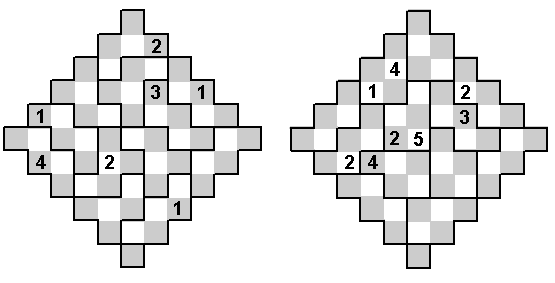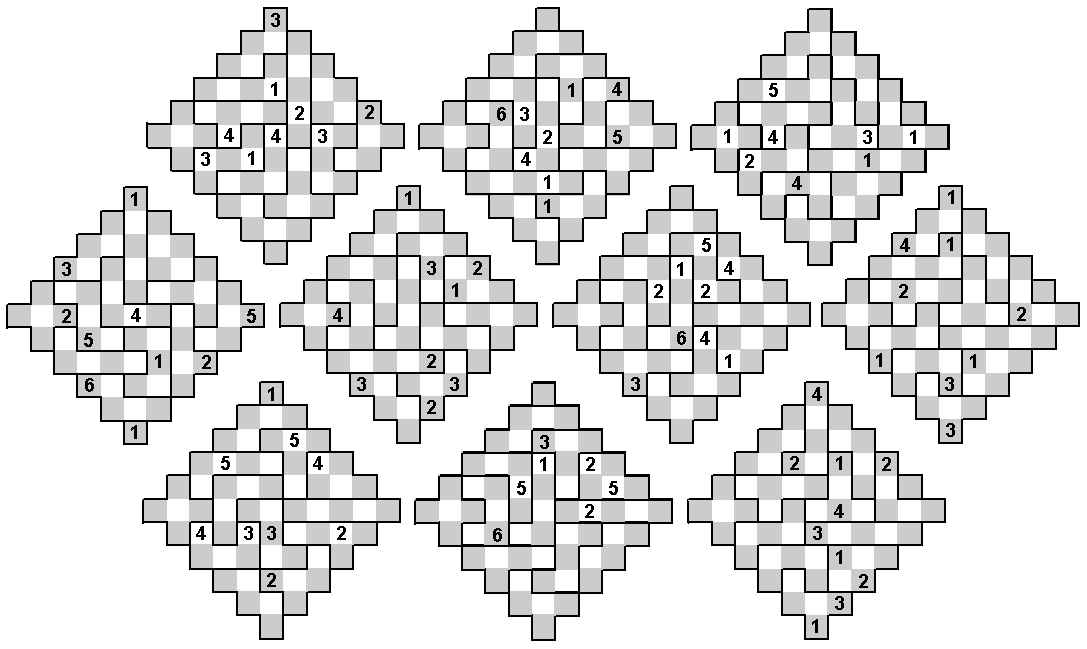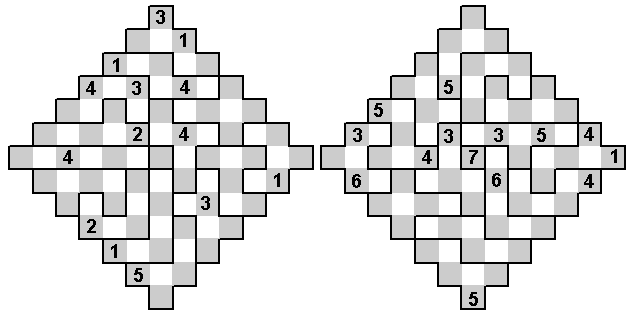Jagged Square Sudoku ProblemsBy a jagged square we mean the type of figure shown below. A jagged square of side n contains n2 +(n-1)2 = 2n(n-1) + 1 squares. Thus a jagged square filled with n-ominoes or (n-1)-ominoes will leave a single square unused. No sudoku type problem can be made by filling with n-ominoes and so these problems use 2n (n-1)-ominoes with a single square remaining.
Here we have the following conditions -
 (a) Each grey diagonal contains the numbers 1 to 6 (b) Each white diagonal contains the numbers 1 to 5 (c) each five square region contains the five of the six numbers 1 to 6.
The first example has twelve copies of a pentomino while the second has six copies each of two pentominoes.Here we again have six copies each of a pair of pentominoes with the conditions.
 (a) Each grey diagonal contains the numbers 1 to 6 (b) Each white diagonal contains the numbers 1 to 5 (c) Each of one set of congruent pieces contains the numbers 1 to 5 (d) Each of the other set of congruent pieces contains five of the six numbers 1 to 6 with a different number omitted from each piece.Using a full set of pentominoes there are ten essentially different solutions to filling the jagged square.None of these has the single square at the centre.Here we have side seven jagged squares filled with hexominoes.
 (a) Each grey diagonal contains the numbers 1 to 7 (b) Each white diagonal contains the numbers 1 to 6 (c) each five square region contains the five of the six numbers 1 to 7.The one-sided pentominoes consist of 18 pieces. If we remove the I-pentomino (the 1x5 rectangle) then the remaining 17 pieces can fill a side seven jagged square in 618 different ways. Here are three examples with the following conditions.
 (a) Each grey diagonal contains the numbers 1 to 7 (b) Each white diagonal contains the numbers 1 to 6 (c) Each pentomino contains five of the numbers 1 to 7Finally, if we add extra white diagonals we get a slightly different type of jagged square. In this example each diagonal (white or grey) contains the numbers 1 to 6 as do each of the hexominoes.